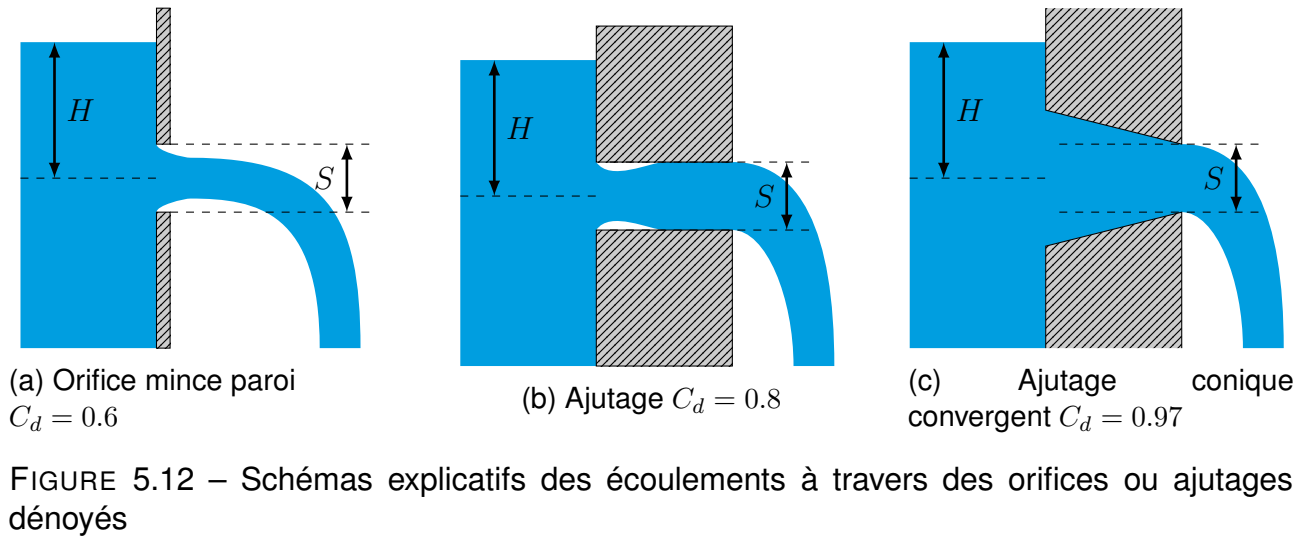# Free orifice formulaExcerpt from CARLIER, M. (1972). Hydraulique générale et appliquée. OCLC : 421635236. Paris : Eyrolles

The general formula for a free orifice or nozzle is as follows (CARLIER, 1972):

$Q = C_d S \sqrt{2g H}$

With:

• $$Q$$ the flow in m3/s;
• $$C_d$$ the discharge coefficient;
• $$S$$ the orifice surface in m2;
• $$g$$ the acceleration of gravity 9.81 m/s2
• $$H$$ The water level measured from the surface of the water to the centre of the orifice in meters.

The area $$S$$ to be considered is the smallest cross-sectional area of the orifice or nozzle (Figure 5.12c). The discharge coefficient $$C_d$$ varies depending on the type of orifice or nozzle. Figure 5.12 shows the most common shapes and discharge coefficients (Source: CARLIER, 1972).